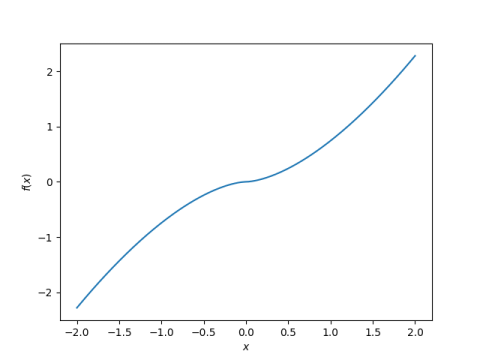# When derivative equals inverse

Is there a function whose derivative is its inverse? In other words, is there a function f that satisfies

f ‘(x) = f-1(x)

for positive x? Indeed there is one given here.

Let φ be the golden ratio (√5 + 1)/2. Then for x > 0 the function

f(x) = φ (x/φ)φ

satisfies our equation. It’s derivative is its inverse. To prove this, calculate

f ‘( f(x) )

and find that it equals x. The reason the golden ratio comes up is that if

f(x) = k (x/k)k

is a solution then k must satisfy

k² – k = 1

and φ is a solution.

Whenever you see one root of a quadratic equation, it’s natural to wonder about the other root. In this case

ψ = -1/φ

is the other root to our quadratic equation.  Could we have used ψ rather than φ? Yes, but the solution would blow up at 0. By using φ, our solution can be extended to allow not just x > 0 but x ≥ 0.

We could also define f for x < 0 using k = φ or k = ψ. But the former blows up as x approaches 0 from the left and the latter does not. So if we use k = ψ to the left of 0 and k = φ to the right, we get a function defined for all real x. That is, we can define

f(x) = φ (x/φ)φ   for x ≥ 0

f(x) = ψ (x/ψ)ψ   for x < 0

and have a continuous function on the whole real line.

We need to be a little careful here about what we mean by raising a negative number to an irrational exponent. To be more explicit we should say

f(x) = φ (x/φ)φ   for x ≥ 0

f(x) = -ψ (-x/ψ)ψ   for x < 0

Here’s a plot:Just to quickly open up a new can of worms before I close this out, you could go back to the definition

f(x) = φ (x/φ)φ

for x > 0 and consider it a function of a complex variable. You could extend it to most of the complex plane, but you’d have to have a branch cut that includes the origin. The construction above wouldn’t work because the function is not analytic at 0. In fact, it’s not twice differentiable at 0.

## 3 thoughts on “When derivative equals inverse”

1. Raúl Martínez

John,

Thanks for the interesting post. But aside from its mathematical beauty, is there an application whence this originates?

Thanks for your blog, which is always worth reading. Keep up the good work.

2. I don’t know of any application.

3. Nathan Hannon

I don’t see what’s going on for x < 0. It seems pretty clear from the graph that, for x < 0, f is negative and increasing, meaning that f(x) is negative and f'(f(x)) is positive; i. e., not equal to x.

In fact, if "inverse" means a two-sided inverse, such a function cannot be defined on the entire real line. If such a function has an inverse, it is injective, so its derivative cannot take on both positive and negative values. But this means that the domain of the function cannot include both positive and negative values.